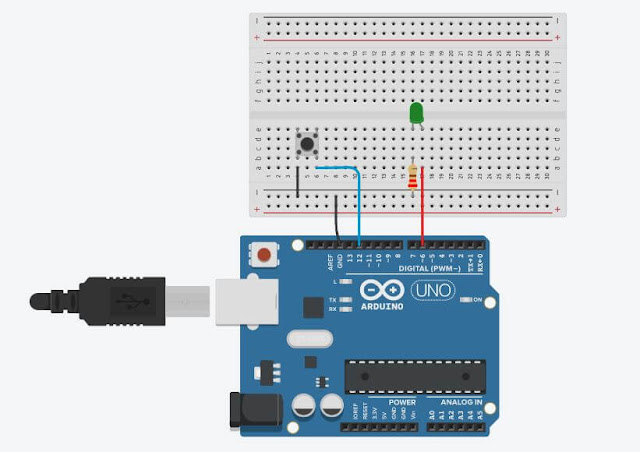## Saturday, September 4, 2021Fig. 1 - Arduino Lesson 4 - Read Pushbutton with digitalRead() function

# Welcome to Lesson 4 - Basic Arduino Course

Today we are going to learn how to read a Pushbutton, which when pressed, the Arduino will read and light an LED indicating that the button was pressed. We will use the digitalRead() function.

## Hardware Required

• Arduino Board
• A Pushbutton
• LED - optional "You can use the onboard LED"
• 220 ohm resistor - optional "You can use the onboard resistor"

## The Circuit

You can use as an example the built-in LED that most Arduino boards have. This LED is connected to a digital pin 13, however, this number may vary from board type to board type.

This constant is LED_BUILTIN and allows you to control the built-in LED easily, and the correspondence of this constant is digital pin 13.

However, you can light an external LED with this sketch, you just need to build the same circuit as the previous lesson, Lesson 3, as we will be using the same circuit, only added from the Microswitch as shown in Figure 2 below,Fig. 2 - Arduino Read a Microswitch with digitalRead  function - tinkercad.com

## The Code

After building the circuit, connect your Arduino board to your computer, launch the Arduino Software (IDE), copy the code below and paste it into your Arduino IDE. But first, let's understand the code line by line.

• First we have the integer declarations, in Line 4 and in Line 5, they are integer constants, as they will not change their parameters, these are the pins that will connect the Push Button and the LED.

• In Line 8, we create a variable that will receive the initial value "0", it will receive the state of the switch depending on whether you press it or not, it will change its value.
 123456789 // Arduino: Lesson 4 - Read Pushbutton with digitalRead() function  // Constants don't change.   // That's why they are used to set the pin numbersconst int buttonPin = 12;    // The number of the pushbutton pinconst int ledPin =  6;          // The number of the LED pin// This variable will change according to the state of the LEDint buttonState = 0;            // Variable for reading the pushbutton status
• After the declarations, we enter the in void setup() function, this function will only be read once at Arduino startup.
Here where we declare for Arduino where we connect our peripherals.

• In Line 11 we use the ledPin declaration as output

• In Line 12 we declare our buttonPin as input Pullup, its means that we are using the Arduino's internal Pullup resistor, so we don't need to use an external resistor together with the switch.
 1...1011121314 // Arduino: Lesson 4 - Read Pushbutton with digitalRead() functionvoid setup() { pinMode(ledPin, OUTPUT); // Initialize digital pin 6 as an output.   pinMode(buttonPin, INPUT_PULLUP);  // Initialize the pushbutton pin as an input pull up}
• After setting the initializations, in Line 15 we will enter the void loop() function.

• In Line 16we get the state of the switch, when pressed, the variable gets "0", and when released, the variable gets "1".

You may be thinking, but it shouldn't be the other way around, when you press it it goes to "1" and when I release it it goes to "0".

Well, remember we use the Arduino's internal Pullup resistor, its means when the switch is released the Pullup resistor leaves the Arduino's gate at HIGH, "1", and when you press the switch it throws the output to the ground, which means we ground output "0" so it goes to LOW.

• In Line 18, we declare a control structure, using the IF conditional, with it we are going to check if the key was pressed, if so, the variable buttonState will receive the value LOW, "0". If released, it will receive the HIGH value "1".
• In Line 19, the digitalWrite function, command activates ledPin to HIGH level, it means that it goes from 0V to 5V, which makes the LED receive the voltage and lit up.

• In Line 20, we have the "else" control structure, that will be triggered if the "if" structure control isn't true.

• In Line 21, the digitalWrite function, command activates ledPin to LOW level, it means that it goes from 5V to 0V, which makes the LED turn off.
 1...151617181920212223 // Arduino: Lesson 4 - Read Pushbutton with digitalRead() functionvoid loop() { // The loop function runs over and over again forever  buttonState = digitalRead(buttonPin);   // Read the state of the pushbutton value  if (buttonState == LOW) {  // Check if pushbutton is pressed. Case is, the buttonState is LOW    digitalWrite(ledPin, HIGH); // Turn LED Turn On  } else {    digitalWrite(ledPin, LOW);  // Turn LED Turn Off  }}

### The complete code is showed in the sketch below!

 123456789101112131415161718192021222324 // Arduino: Lesson 4 - Read Pushbutton with digitalRead() function// Constants don't change.    // That's why they are used to set the pin numbersconst int buttonPin = 12;    // The number of the pushbutton pinconst int ledPin =  6;          // The number of the LED pin// This variable will change according to the state of the LEDint buttonState = 0;            // Variable for reading the pushbutton statusvoid setup() {                                // This function is called once when the program starts pinMode(ledPin, OUTPUT); // Initialize digital pin 6 as an output.   pinMode(buttonPin, INPUT_PULLUP);   // Initialize the pushbutton pin as an input pull up}void loop() {                                      // The loop function runs over and over again forever  buttonState = digitalRead(buttonPin);   // Read the state of the pushbutton value  if (buttonState == HIGH) {                     // Check if pushbutton is pressed. Case is, the buttonState is HIGH    digitalWrite(ledPin, HIGH);                 // Turn LED Turn On  } else {    digitalWrite(ledPin, LOW);                  // Turn LED Turn Off  }}//------------------------------------- www.elcircuits.com --------------------------------------------